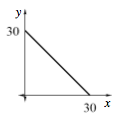### Home > CCA2 > Chapter 1 > Lesson 1.1.2 > Problem1-19

1-19.

Jill needs to cut a piece off of a $30$-foot length of lumber. Create multiple representations ( $x → y$ table, graph, and equation) for the function with $x$-values that are the length of the piece Jill cuts off and $y$-values that are the length of the piece that is left over. Which representation best portrays the situation? Why? Explain.

Make a table.

What input and output represent the length of the lumber before she cuts it?

 $x$ $y$ $0$ $1$ $6$ $20$ $30$ $29$ $24$ $10$

Draw a graph. What is the slope?What is the $y$-intercept? What is the equation?

$y = 30 − x$

Decide which representation best shows that when she cuts off a longer piece, the remaining piece is shorter, and explain your reasoning.